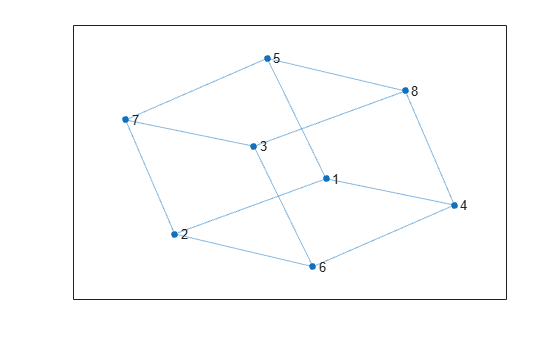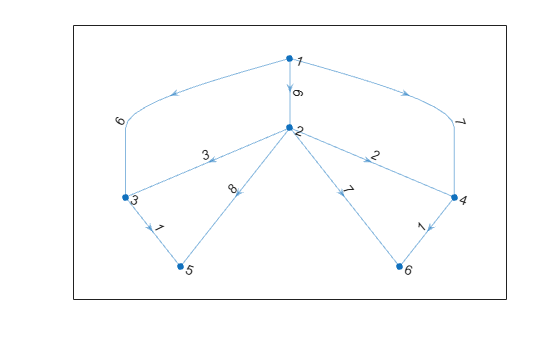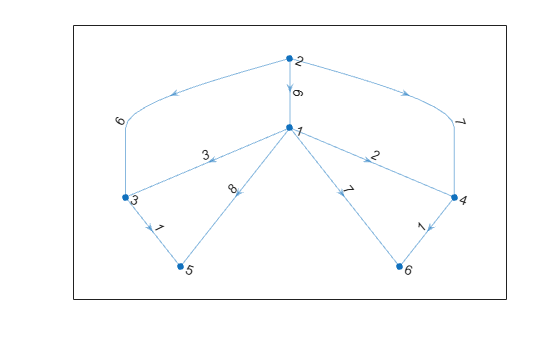# reordernodes

Reorder graph nodes

## Syntax

``H = reordernodes(G,order)``
``````[H,idx] = reordernodes(G,order)``````

## Description

example

````H = reordernodes(G,order)` reorders the nodes in graph `G` according to `order`. This reordering corresponds to a symmetric permutation of the adjacency matrix of `G`.```

example

``````[H,idx] = reordernodes(G,order)``` also returns the permutation vector for the edge indices, `idx`. For example, if `G.Edges` has a variable `Weight`, then `H.Edges.Weight == G.Edges.Weight(idx)`.```

## Examples

collapse all

Create and plot a graph.

```s = [1 1 1 2 5 3 6 4 7 8 8 8]; t = [2 3 4 5 3 6 4 7 2 6 7 5]; G = graph(s,t); plot(G)```Reorder the graph nodes using a permutation vector.

```order = [7 2 3 4 8 1 5 6]; G = reordernodes(G,order); plot(G)```Create and plot a weighted graph.

```s = [1 1 1 2 2 2 2 3 4]; t = [3 4 2 3 4 5 6 5 6]; weights = [6 7 6 3 2 8 7 1 1]; G = digraph(s,t,weights); plot(G,'EdgeLabel',G.Edges.Weight)```Reorder the graph nodes based on the out-degree, such that node 1 has the largest out-degree.

`[~,order] = sort(outdegree(G),'descend')`
```order = 6×1 2 1 3 4 5 6 ```
```[H,idx] = reordernodes(G,order); plot(H,'EdgeLabel',H.Edges.Weight)````idx` describes the permutation of the rows in `G.Edges`. Confirm this correspondence using the `Weight` variable.

`isequal(H.Edges.Weight, G.Edges.Weight(idx))`
```ans = logical 1 ```

## Input Arguments

collapse all

Input graph, specified as either a `graph` or `digraph` object. Use `graph` to create an undirected graph or `digraph` to create a directed graph.

Example: `G = graph(1,2)`

Example: `G = digraph([1 2],[2 3])`

Node order, specified as node indices or node names. `order` specifies a symmetric permutation of the adjacency matrix of `G`. If ```A = adjacency(G)```, then `A(order,order)` produces `adjacency(H)`.

`order` can be one of:

• Numeric vector of node indices, such as ```[1 3 2]```.

• A cell array of character vectors or string array of node names, such as `{'A' 'C' 'B'}` or ```["A" "C" "B"]```.

Example: `H = reordernodes(G,[3 1 2])`

## Output Arguments

collapse all

Output graph, returned as a `graph` or `digraph` object. `H` contains the same `Nodes` and `Edges` properties as `G`, but with the rows of `H.Nodes` and `H.Edges` permuted:

• `H.Nodes` is the same as `G.Nodes(order,:)`.

• `H.Edges` is similar to `G.Edges(idx,:)`, except that the nodes are renumbered.

Permutation vector of edge indices, returned as a vector. The values in `idx` describe the permutation of the rows in `G.Edges`.